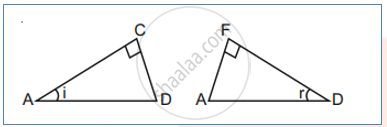# Define the Term Wavefront. Using Huygen’S Wave Theory, Verify the Law of Reflection. - Physics

Define the term wavefront. Using Huygen’s wave theory, verify the law of reflection.

#### Solution

In a region where the wave is propagating, the geometrical structure formed by joining all the points which are vibrating in same (absolute) phase are said to be wavefront Reflection of plane wave :Consider a plane wave ABC incident at an angle ‘i’ on a reflecting surface ‘AD’. If v represents the speed of the wave in medium Δt is the time taken to move from ABC to B1C1 and also from B1C1 to point ‘D’. So, at the time when a disturbance of point ‘C’ reaches to point ‘D’ disturbance of point ‘A’ must have formed a hemispherical shape of radius 2vΔt and at point ‘B1’ it must have formed a hemispherical shape of radius vΔt. Drawing a common tangent from point ‘D’ to both of the above mentioned spherical envelopes we shall have DEF as reflected wavefront. Now, in ΔACD and ΔDFAAnd ∠ACD = ∠DFA = 90°

so by RHS rule, both the triangles are congruent. Hence each corresponding parameter must be the same. Hence ∠CAD = i (angle of incidence) Must be the same as ∠FDA = r (angle of reflection) ∠i = ∠r

Concept: Huygens' Principle
Is there an error in this question or solution?